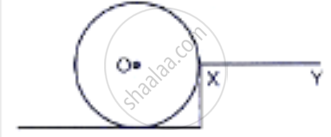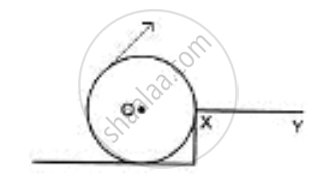Share

# The Adjacent Diagram Shows a Heavy Roller, with Its Axle at O. Which Its Axle at O. Which is to Be Raised on a Pavement Xy by Applying a Minimum Possible Force. - ICSE Class 10 - Physics

#### Question

The adjacent diagram shows a heavy roller, with its axle at O. which its axle at O. which is to be raised on a pavement XY by applying a minimum possible force. show by an arrow on the diagram the point of application and the direction in which the force should be applied.#### SolutionForce F should be provided in the direction as shown in the diagram.

Is there an error in this question or solution?
Solution The Adjacent Diagram Shows a Heavy Roller, with Its Axle at O. Which Its Axle at O. Which is to Be Raised on a Pavement Xy by Applying a Minimum Possible Force. Concept: Concept of Force.
S